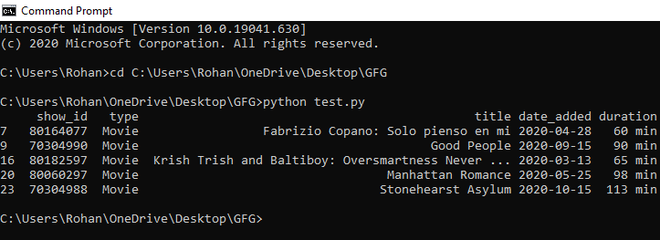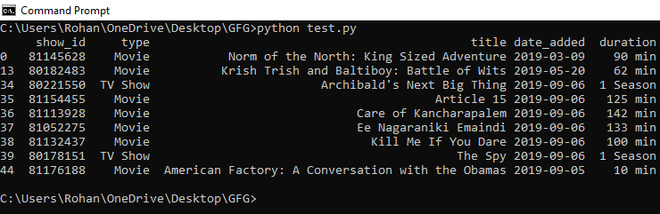# How to Filter DataFrame Rows Based on the Date in Pandas?

Filtering a DataFrame rows by date selects all rows which satisfy specified date constraints, based on a column containing date data. For instance, selecting all rows between March 13, 2020, and December 31, 2020, would return all rows with date values in that range.

Use DataFrame.loc() with the indexing syntax [condition] to select only the rows from DataFrame which satisfy condition.Define condition to check if the date column in DataFrame is within the constraints.It will create a boolean array for all the rows. Only rows having true value will be printed.

Approach:

• Convert the data column into date format supported by python
• Filter the rows on the basis of date
• Access rows using .loc() function and store them in dataframe.

Example 1:

## Python3

 `# import pandas library ` `import` `pandas as pd ` ` `  `# load csv file ` `df ``=` `pd.read_csv( ` `    ``"C:\\Users\\Rohan\\OneDrive\\Desktop\\GFG\\netflix_titles.csv"``) ` ` `  `# convert date column into date format ` `df[``'date_added'``] ``=` `pd.to_datetime(df[``'date_added'``]) ` ` `  `# filter rows on the basis of date ` `newdf ``=` `(df[``'date_added'``] > ``'01-03-2020'``) & (df[``'date_added'``] <``=` `'31-12-2020'``) ` ` `  `# locate rows and access them using .loc() function ` `newdf ``=` `df.loc[newdf] ` ` `  `# print dataframe ` `print``(newdf) `

Output:All the movies between date 13-Mar-2020 and 31-Dec-2020 will be printed.

Example 2:

## Python3

 `# import pandas library ` `import` `pandas as pd ` ` `  `# load csv file ` `df ``=` `pd.read_csv( ` `    ``"C:\\Users\\Rohan\\OneDrive\\Desktop\\GFG\\netflix_titles.csv"``) ` ` `  `# convert date column into date format ` `df[``'date_added'``] ``=` `pd.to_datetime(df[``'date_added'``]) ` ` `  `# filter rows on the basis of date ` `newdf ``=` `(df[``'date_added'``] > ``'01-01-2019'``) & (df[``'date_added'``] <``=` `'31-12-2019'``) ` ` `  `# locate rows and access them using .loc() function ` `newdf ``=` `df.loc[newdf] ` ` `  `# print dataframe ` `print``(newdf) `

Output:The above output prints all the movies added on Netflix in the year 2019.

Whether you're preparing for your first job interview or aiming to upskill in this ever-evolving tech landscape, GeeksforGeeks Courses are your key to success. We provide top-quality content at affordable prices, all geared towards accelerating your growth in a time-bound manner. Join the millions we've already empowered, and we're here to do the same for you. Don't miss out - check it out now!

Previous
Next